Saturday, September 26, 2009

Reference Angle

Reference angles are used to determine the values of the trigonometric functions in the second, third and fourth quadrants, in particular, for the "nice" angles. The reference angle for an angle θ is the smallest angle φ from the (positive or negative) x-axis to the terminal ray of the angle θ.

For an angle θ in the second quadrant the reference angle φ is the remaining angle needed to complete a straight angle, that is, π radians or 180°. Thus θ + φ = π or θ + φ = 180°, and so

φ = π - θ or φ = 180° - θ.For an angle θ in the third quadrant the reference angle φ is the angle that must be subtracted from θ to leave a straight angle, that is, π radians or 180°. Thus θ - φ = π or θ - φ = 180°, and so

φ = θ - π or φ = θ - 180°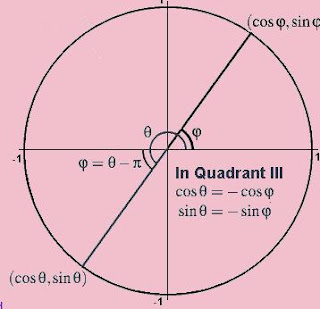For an angle θ in the fourth quadrant the reference angle φ is the remaining angle needed to complete a full circle angle, that is, 2π radians or 360°. Thus θ + φ = 2π or θ + φ = 360°, and so

φ = 2π - θ or φ = 360° - θ.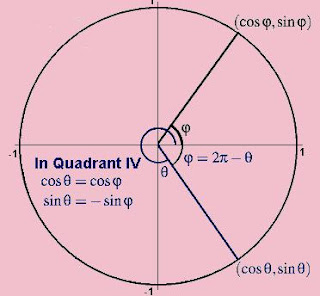Friday, September 25, 2009

Signs of sine, cosine and tangent, by Quadrant

The definition of the trigonometric functions cosine and sine in terms the coordinates of points lying on the unit circle tell us the signs of the trigonometric functions in each of the four quadrants, based on the signs of the x and y coordinates in each quadrant.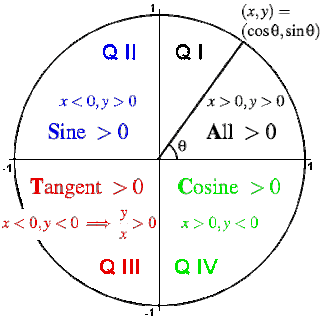For an angle in the first quadrant the point P has positive x and y coordinates. Therefore: In Quadrant I, cos(θ) > 0, sin(θ) > 0 and tan(θ) > 0 (All positive).

For an angle in the second quadrant the point P has negative x coordinate and positive y coordinate. Therefore: In Quadrant II, cos(θ) < 0, sin(θ) > 0 and tan(θ) < 0 (Sine positive).

For an angle in the third quadrant the point P has negative x and y coordinates. Therefore: In Quadrant III, cos(θ) < 0, sin(θ) < 0 and tan(θ) > 0 (Tangent positive).

For an angle in the fourth quadrant the point P has positive x coordinate and negative y coordinate. Therefore: In Quadrant IV, cos(θ) > 0, sin(θ) < 0 and tan(θ) < 0 (Cosine positive).

The quadrants in which cosine, sine and tangent are positive are often remembered using a favorite mnemonic.
One example: All Students Take Calculus.

Unit CircleSo... what is a Unit Circle?

A unit circle is a circle with a radius of one (a unit radius). In trigonometry, the unit circle is centered at the origin.

For the point (x,y) in Quadrant I, the lengths x and y become the legs of a right triangle whose hypotenuse is 1.

In the diagram above, we're measuring the angle θ between the x-axis of the Cartesian plane and a line that extends from the origin. Now, here's the really interesting thing; the sine of the angle is equal to the y-coordinate of the point on the unit circle where the line crosses, and the cosine of the angle is equal to the x-coordinate. This is true for any line extending from the origin.

Why is this? Well, the line segment from the origin to the point where it crosses the unit circle forms the hypotenuse of a right-angled triangle. Because the radius of the circle is 1, the length of the hypotenuse is likewise 1. SOHCAHTOA's rules then boil down to:

Sin θ = Opposite

Cos θ = Adjacent

Tan θ = Opposite/Adjacent

In other words:

Sin θ = y

Cos θ = x

Tan θ = y/x

Monday, September 14, 2009

Common Tangent To A Pair Of Circles

Common tangents are lines or segments that are tangent to more than one circle at the same time.

The possibility of common tangents is closely linked to the mutual position of circles.

If two circles touch inside, the two internal tangents vanish and the two external ones become a single tangent.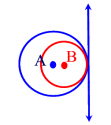If two circles intersect, the common tangent is replaced by a common secant, whence there are only two external tangents.If two circles touch each other outside, the two internal tangents coincide in a common tangent, thus there are three common tangents.If two circles are separate, there are four common tangents, two inside and two outside.Tangent Of A Circle.

I started the chapter on circle by introducing the concept of tangent to a circle.

A tangent to a circle has two defining properties

* A tangent touches a circle in exactly one point. This point is called point of tangency.
* The tangent intersects the circle's radius at a 90° angle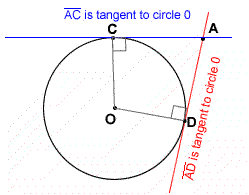Watch Video on Tangent Line

Properties of two tangents to a circleIf two tangents lines are drawn namely AP and BP, they will intersect at a point, P. The properties of the two tangent lines are listed below :

1. CA = CB
2. AP = BP
3. Angle ACP = Angle BCP
4. Angle Angle APC = Angle BPC
5. Angle CAP and angle CBP are both right angles
6. Triangle CAP and BCP are congruent

Sunday, September 13, 2009

Blogging Again

It's been quite a while since my last update. It's not that I'm been so busy, it's just that the drive to write was not there. Writing does not come easily for me, I guess its just not my cup of tea.

School reopened on the 1st of September 2009. I managed to finished off the Earth As A Sphere chapter with my 5KA class before they start their trial exam on the 8th of September. I really hope that my girls will do well in this exam. I'm really amazed at the improvements shown by a number of girls in that class. Good Luck to all of you.

My 4SA class did quite well in the August test. Only 2 students were not able to score an A for the test. I'll have to monitor the two girls more closely. I really need to speed up on my teaching coz I just realized that I only have two weeks after the Hari Raya break before the final exam starts. Last week, I missed the Wednesday class coz I had to go for a meeting in Shah Alam. This week I will again miss the Wednesday class due to another meeting in BTP. Thankfully Datin Lee is around to cover for me. I really feel guilty when I had to miss my class but being the ICT coordinator of my school, I'm frequently called for meetings. I wish someone will take over my job coz I'd rather be teaching than doing all the administrative work.